# Selina Concise Mathematics Class 7 ICSE Solutions Chapter 21 Data Handling

## Selina Concise Mathematics Class 7 ICSE Solutions Chapter 21 Data Handling

Selina Publishers Concise Mathematics Class 7 ICSE Solutions Chapter 21 Data Handling

### Data Handling Exercise 21A – Selina Concise Mathematics Class 7 ICSE Solutions

Question 1.
Consider the following numbers :
68, 76, 63, 75, 93, 83, 70, 115, 82, 105, 90, 103, 92, 52, 99, 73, 75, 63, 77 and 71.
(i) Arrange these numbers in ascending order.
(ii) What the range of these numbers?
Solution:Question 2.
Represent the following data in the form of a frequency distribution table :
16, 17, 21, 20, 16, 20, 16, 18, 17, 21, 17, 18, 19, 17, 15, 15, 19, 19, 18, 17, 17, 15, 15, 16, 17, 17, 19, 18, 17, 16, 15, 20, 16, 17, 19, 18, 19, 16, 21 and 17.
Solution:Question 3.
A die was thrown 20 times and following scores were recorded.
2, 1, 5, 2, 4, 3, 6, 1, 4, 2, 5, 1, 6, 2, 6, 3, 5, 4, 1 and 3.
Prepare a frequency table for the scores.
Solution:Question 4.
Following data shows the weekly wages (in ₹) of 10 workers in a factory.
3500, 4250, 4000, 4250, 4000, 3750, 4750, 4000, 4250 and 4000
(i) Prepare a frequency distribution table.
(ii) What is the range of wages (in ₹)?
(iii) How many workers are getting the maximum wages?
Solution:Question 5.
The marks obtained by 40 students of a class are given below :
80, 10, 30, 70, 60, 50, 50, 40, 40, 20, 40, 90, 50, 30, 70, 10, 60, 50, 20, 70, 70, 30, 80, 40,20, 80, 90, 50, 80, 60, 70, 40, 50, 60, 90, 60, 40, 40, 60 and 60
(i) Construct a frequency distribution table.
(ii) Find how many students have marks equal to or more than 70?
(iii) How many students obtained marks below 40?
Solution:Question 6.
Arrange the following data in descending order:
3.3, 3.2, 3.1, 3.7, 3.6, 4.0, 3.5, 3.9, 3.8, 4.1, 3.5, 3.8, 3.7, 3.9 and 3.4.
(i) Determine the range.
(ii) How many numbers are less than 3.5?
(iii) How many numbers are 3.8 or above?
Solution:### Data Handling Exercise 21B – Selina Concise Mathematics Class 7 ICSE Solutions

Question 1.
Find the mean of 53, 61, 60, 67 and 64.
Solution:Question 2.
Find the mean of first six natural numbers.
Solution:Question 3.
Find the mean of first ten odd natural numbers.
Solution: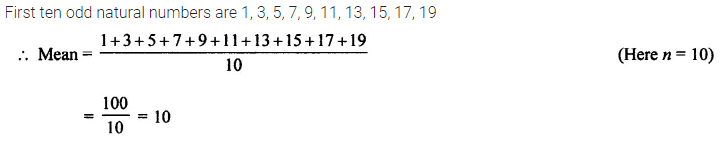Question 4.
Find the mean of all factors of 10.
Solution: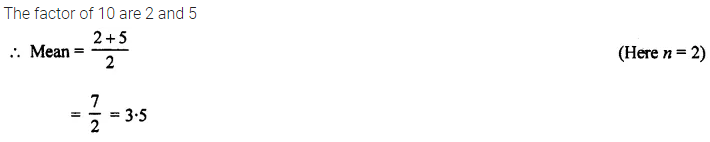Question 5.
Find the mean of x + 3, x + 5, x + 7, x + 9 and x + 11.
Solution:Question 6.
If different values of variable x are 19.8,15.4,13.7,11.71,11.8, 12.6,12.8,18.6,20.5 and 2.1, find the mean.
Solution:Question 7.
The mean of a certain number of observations is 32. Find the resulting mean, if each observation is,
(i) increased by 3
(ii) decreased by 7
(iii) multiplied by 2
(iv) divided by 0.5
(v) increased by 60%
(vi) decreased by 20%
Solution: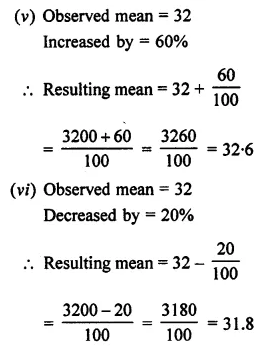Question 8.
The pocket expenses (per day) of Anuj, during a certain week, from monday to Saturday were ₹85.40, ₹88.00, ₹86.50, ₹84.75, ₹82.60 and ₹87.25. Find the mean pocket expenses per day.
Solution:Question 9.
If the mean of 8, 10, 7, x + 2 and 6 is 9, find the value of x.
Solution:Question 10.
Find the mean of first six multiples of 3.
Solution:Question 11.
Find the mean of first five prime numbers.
Solution:Question 12.
The mean of six numbers :x-5,x- 1, x, x + 2, x + 4 and x + 12 is 15. Find the mean of first four numbers.
Solution: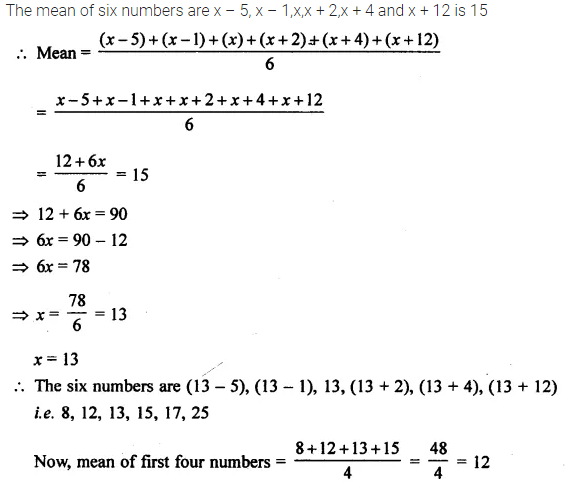Question 13.
Find the mean of squares of first five whole numbers.
Solution:Question 14.
If the mean of 6, 4, 7, p and 10 is 8, find the value of p.
Solution:Question 15.
Find the mean of first six multiples of 5.
Solution:Question 16.
The rainfall (in mm) in a city on 7 days of a certain week is recorded as followsFind the total and average (mean) rainfall for the week.
Solution:Question 17.
The mean of marks scored by 100 students was found to be 40, later on it was discovered that a score of 53 was misread as 83. Find the correct mean.
Solution: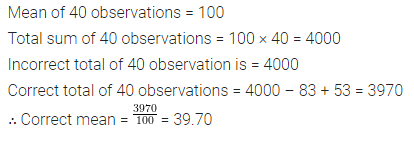Question 18.
The mean of five numbers is 27. If one number is excluded, the mean of remaining numbers is 25. Find the excluded number.
Solution:Question 19.
The mean of 5 numbers is 27. If one new number is included, the new mean is 25. Find the included number.
Solution:Question 20.
Mean of 5 numbers is 20 and mean of other 5 numbers is 30. Find the mean of all the 10 numbers taken together.
Solution: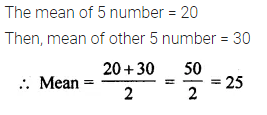Question 21.
Find the median of:
(i) 5,7, 9, 11, 15, 17,2, 23 and 19
(ii) 9, 3, 20, 13, 0, 7 and 10
(iii) 18, 19, 20, 23, 22, 20, 17, 19, 25 and 21
(iv) 3.6, 9.4, 3.8, 5.6, 6.5, 8.9, 2.7, 10.8, 15.6, 1.9 and 7.6.
Solution: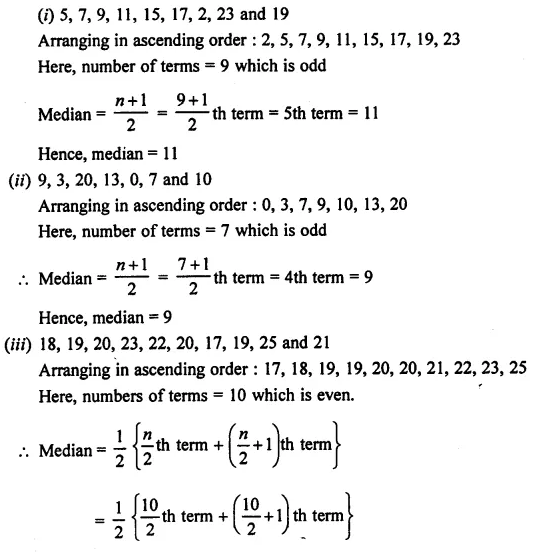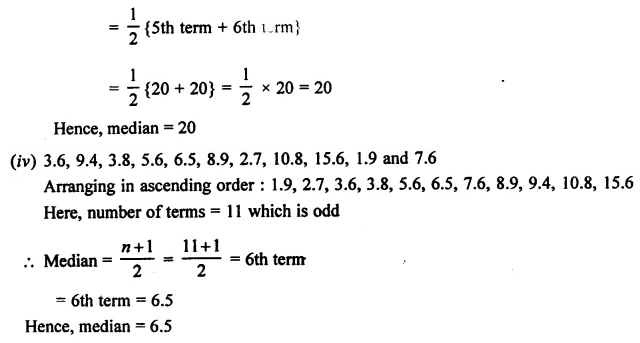Question 22.
Find the mean and the mode for the following data :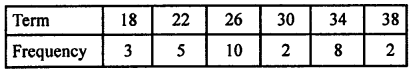Solution:Question 23.
Find the mode of:
(i) 5, 6, 9, 13, 6, 5, 6, 7, 6, 6, 3
(ii) 7, 7, 8, 10, 10, 11, 10, 13, 14
Solution:Question 24.
Find the mode of :Solution:Question 25.
The heights (in cm) of 8 girls of a class are 140,142,135,133,137,150,148 and 138 respectively. Find the mean height of these girls and their median height.
Solution:Question 26.
Find the mean, the median and the mode of:
(i) 12, 24, 24, 12, 30 and 12
(ii) 21, 24, 21, 6, 15, 18, 21, 45, 9, 6, 27 and 15.
Solution: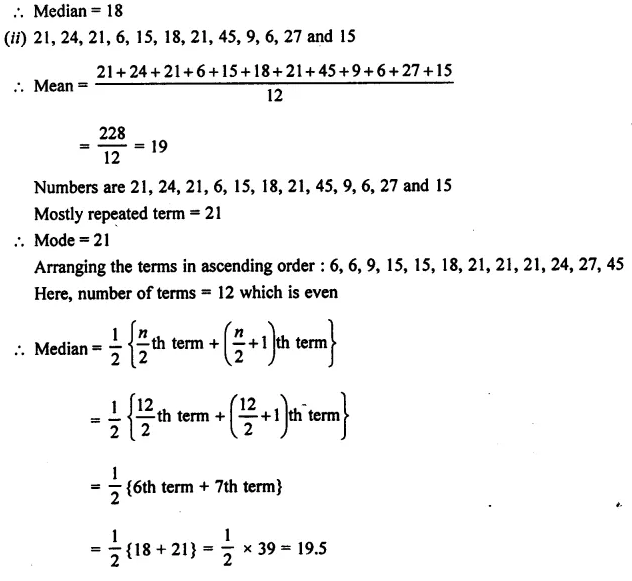Question 27.
The following table shows the market positions of some brands of soap.
Draw a suitable bar graph :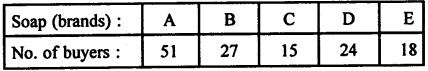Solution:Question 28.
The birth rate per thousand of different countries over a particular period of time is shown below.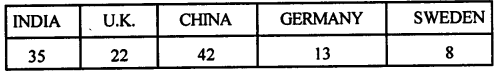Solution: